Physics
Easy

Question

# An object is moving at a uniform speed in a counter clockwise direction in a uniform circular motion. At the location shown, the centripetal force stops acting on the object. Which path will the object follow?

##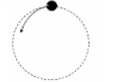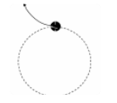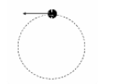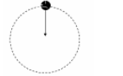Hint:

## The correct answer is:Physics

Physics

Physics

### A girl is sitting in a merry-go-round.  The time period of circular motion of the merry-go-round is 8 sec and it forms a circle of 40 m radius. What is the speed of rotation?Physics

Physics

Physics

### What is the direction of the velocity at point mentioned if the ball is revolving anticlockwise?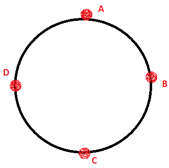At point BPhysics

Physics

Physics

### The time taken for one complete circle is called the _____.Physics

Physics

Physics

### A boy moves a tied stone above his head in circles, what is the time period of circular motion if it forms a circle of 20 cm radius. The speed of rotation is 23 m/s.Physics

Physics

Physics

### What is the direction of the velocity at point mentioned if the ball is revolving clockwise.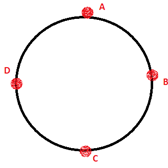At point C#### With Turito Foundation.#### Get an Expert Advice From Turito.